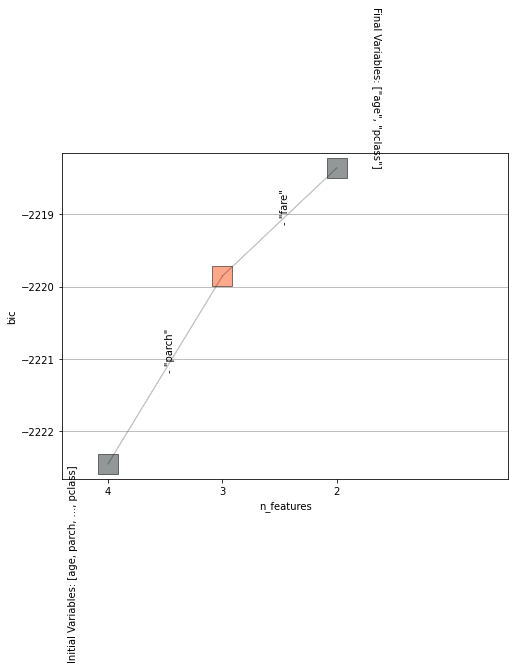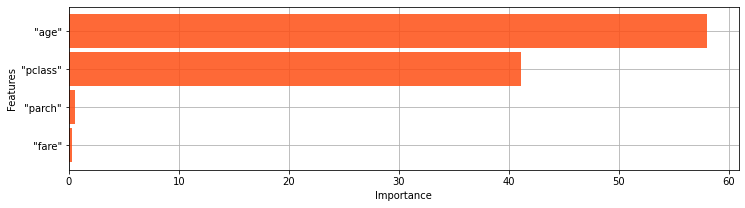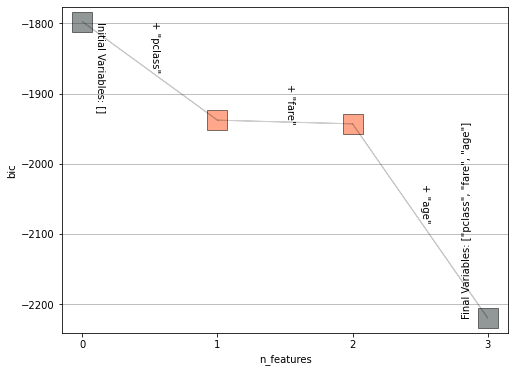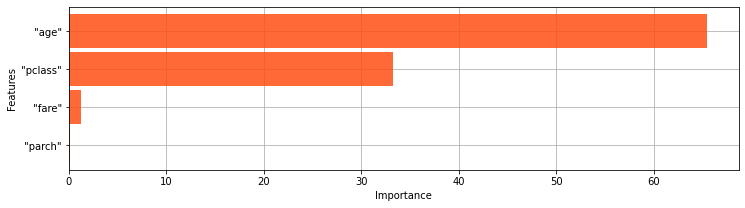# stepwise¶

In [ ]:
```stepwise(estimator,
input_relation: (str, vDataFrame),
X: list,
y: str,
criterion: str = "bic",
direction: str = "backward",
max_steps: int = 100,
criterion_threshold: int = 3,
drop_final_estimator: bool = True,
x_order: str = "pearson",
print_info: bool = True,
show: bool = True,
ax=None,
**style_kwds,)
```

Uses the stepwise algorithm to find the most suitable number of features when fitting the estimator.

### Parameters¶

Name Type Optional Description
estimator
object
Vertica estimator having a fit method and a DB cursor.
input_relation
str / vDataFrame
Input Relation.
X
list
List of the predictor columns.
y
str
Response Column.
criterion
str
Criterion used to evaluate the model.
• aic : Akaike’s Information Criterion.
• bic : Bayesian Information Criterion.
direction
str
How to start the stepwise search. Can be done 'backward' or 'forward'.
max_steps
int
The maximum number of steps to be considered.
criterion_threshold
int
Threshold used when comparing the models criterions. If the difference is lesser than the threshold then the current 'best' model is changed.
drop_final_estimator
bool
If set to True, the final estimator will be dropped.
x_order
str
How to preprocess X before using the stepwise algorithm.
• pearson : X is ordered based on the Pearson's correlation coefficient.
• spearman : X is ordered based on the Spearman's correlation coefficient.
• random : Shuffles the vector X before applying the stepwise algorithm.
• none : Does not change X order.
print_info
bool
If set to True, prints the model information at each step.
show
bool
If set to True, the Stepwise graphic will be drawn.
ax
Matplotlib axes object
The axes to plot on.
**style_kwds
any
Any optional parameter to pass to the Matplotlib functions.

### Returns¶

tablesample : An object containing the result. For more information, see utilities.tablesample.

### Example¶

In :
```from verticapy.learn.linear_model import LogisticRegression
model = LogisticRegression(name = "public.LR_titanic",
tol = 1e-4,
max_iter = 100,
solver = 'Newton')

from verticapy.learn.model_selection import stepwise

# backward
stepwise(model,
input_relation = "public.titanic",
X = ["age", "fare", "parch", "pclass",],
y = "survived",)
```
```Starting Stepwise
```
```[Model 0] bic: -2222.4553063512712; Variables: ['"age"', '"parch"', '"fare"', '"pclass"']
[Model 1] bic: -2219.8559430056216; (-) Variable: "parch"
[Model 2] bic: -2218.3600781845307; (-) Variable: "fare"

Selected Model

[Model 2] bic: -2218.3600781845307; Variables: ['"age"', '"pclass"']
```
Out:bic change variable importance 0 -2222.4553063512712 [null] [null] 0.0 1 -1943.6797392350866 + "age" 58.01108831878302 2 -2219.8559430056216 - "parch" 0.5409078642614441 3 -2218.3600781845307 - "fare" 0.31127816238321365 4 -2020.6752155831307 + "pclass" 41.136725654572324
Rows: 1-5 | Columns: 6In :
```# forward
stepwise(model,
input_relation = "public.titanic",
X = ["age", "fare", "parch", "pclass",],
y = "survived",
direction = "forward",)
```
```Starting Stepwise
```
```[Model 0] bic: -1797.4537281723472; Variables: []
[Model 1] bic: -1937.9168392321783; (+) Variable: "pclass"
[Model 2] bic: -1943.1918759949126; (+) Variable: "fare"
[Model 3] bic: -2219.8559430056216; (+) Variable: "age"

Selected Model

[Model 3] bic: -2219.8559430056216; Variables: ['"pclass"', '"fare"', '"age"']
```
Out:features bic change variable importance 0 [] -1797.4537281723472 [null] [null] 0.0 1 ['pclass'] -1937.9168392321783 + "pclass" 33.21504058447908 2 ['pclass', 'fare'] -1943.1918759949126 + "fare" 1.2473777551759295 3 ['pclass', 'fare', 'parch'] -1943.6797392350866 - "parch" 0.11536407815395282 4 ['pclass', 'fare', 'age'] -2219.8559430056216 + "age" 65.42221758219104
Rows: 1-5 | Columns: 6